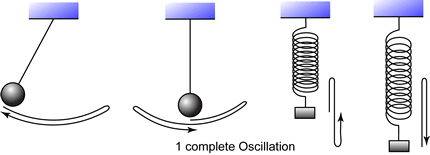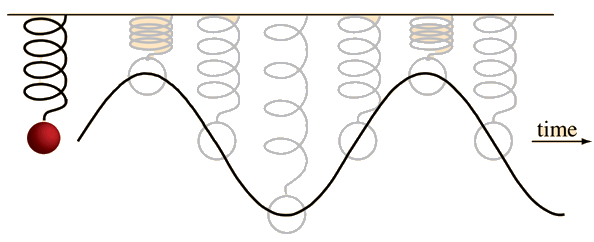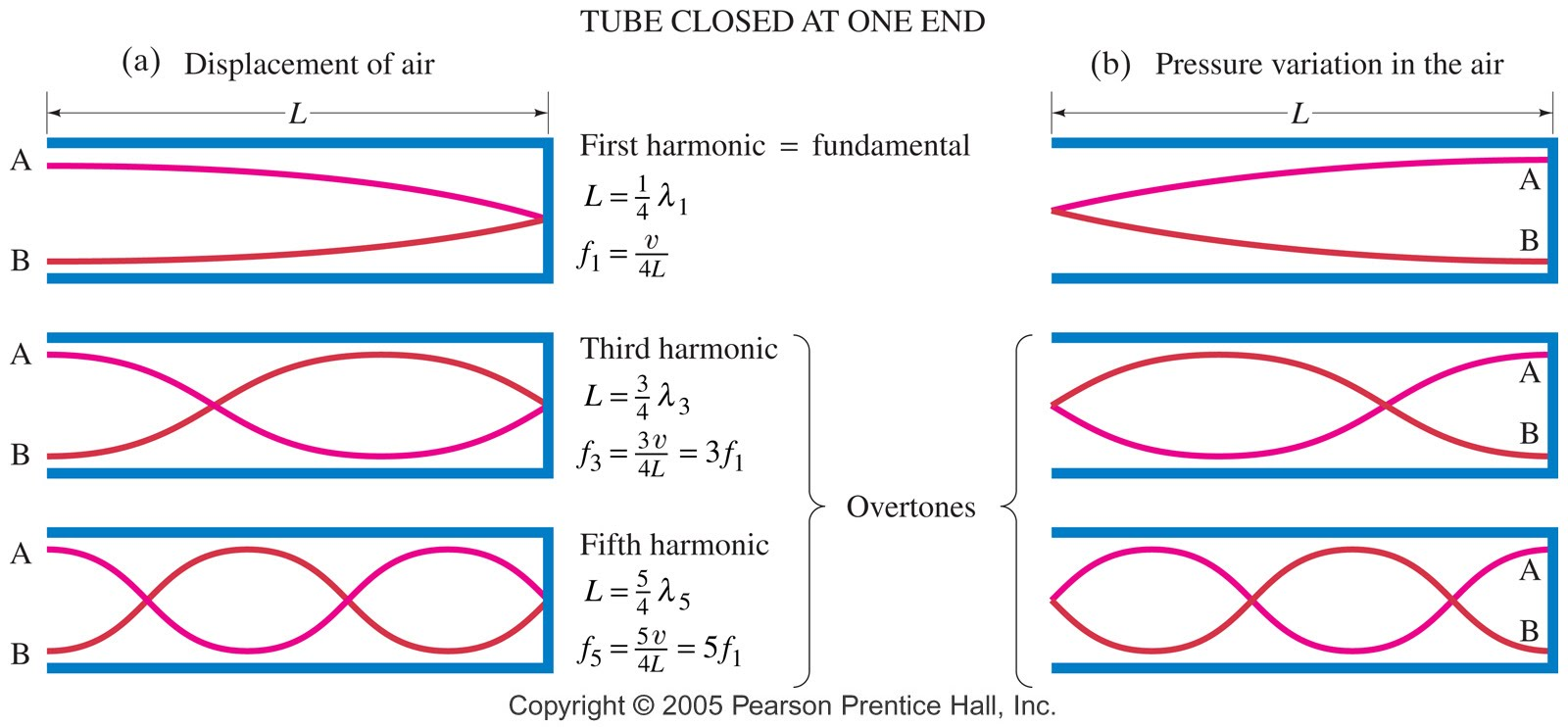# What do you need to know about Oscillations and Waves?

## 12 Aug What do you need to know about Oscillations and Waves?

a

a

Thermal physics provided the transition from macroscopic physics to microscopic physics, and electricity is all about electrons, so why are we backtracking now to talk about bouncing springs and wiggling strings?

It’s because waves are going to be essential, ultimately, to our understanding of the inner workings of the atom. Bohr’s earliest thoughts about the atom involved viewing electrons as little mass/spring systems – “harmonic oscillators” – inside the atom. Later he would switch to a model in which the electrons orbit the nucleus.  Orbits are circular motion, and circular motion is intimately related to simple harmonic motion.  It all ties together!

###### Key Points for IB Students
• F = -kx or a = -kx are the defining equations of simple harmonic motion.  Make sure you know what these graphs look like (straight line, negative slope, through origin)
• Sound is a longitudinal wave
• Electromagnetic waves (light) are the only type that need no medium.
• Light must be a transverse wave, because we can polarize it
• Know how to derive velocity = frequency * wavelength
• Be sure you understand transverse and longitudinal waves
• Be able to use and sketch graphs of displacement vs time AND displacement vs position.  From a graph of displacement vs position, you can get wavelength but not period.  From a graph vs time you can get period but not wavelength.  This is a common source of trouble for students.• A graph of displacement vs time or position looks the same regardless of whether the wave is transverse or longitudinal.  It’s only the direction of the displacement that is different.  For transverse, displacement is perpendicular to the direction the wave moves.  For longitudinal, displacement is parallel to direction of travel of the wave.
• Energy of a wave is proportional to square of amplitude (because energy in SHM is proportional to square of displacement, as we see in energy of a spring = 1/2kx^2)

###### Oscillations and Simple Harmonic Motion (4.1)

a

Did you ever wonder why pi shows up in so many places where you might not expect it? One reason is that simple harmonic motion is related to circular motion.  Pi is not just about circles, it’s about oscillations, and oscillations are everywhere, so pi is everywhere.

aaWatch the following video!

a

a

###### Wave Characteristics (4.3)

a

a) Wavefronts

b) Polarization

Simulator for polarized light passing through polarized film:  http://tutor-homework.com/Physics_Help/polarized_light.html

Watch this Youtube video showing use of polarized light to identify stress in molded plastic

c) Superposition

Good animation of superposition of waves  http://www.acs.psu.edu/drussell/Demos/superposition/superposition.html

###### Wave Behavior (4.4)

a

a) Refraction

Simulator for refraction, critical angle, and total internal reflection: http://phet.colorado.edu/en/simulation/bending-light

a

b) Diffraction

• Single slit diffraction simulator   This sim allows you to see how slit width and wavelength affect the diffraction pattern:  http://www.walter-fendt.de/ph14e/singleslit.htm
• Double slit diffraction demonstration by Veritasium.  Real life demonstration of Young’s famous experiment that “proved” light is a wave

• Double source interference simulator.  This is not an example of diffraction but it does give you a very good picture of what the interference pattern looks like when you have two sources of waves (Since slits are like independent sources, this is the same pattern you get when studying double slit diffraction) http://phet.colorado.edu/en/simulation/sound

###### Standing Waves (4.5)

Wave on string demo showing multiple harmonics with a vibrating string

a) Understanding standing waves as superposed reflections: http://www.acs.psu.edu/drussell/Demos/superposition/superposition.html

b) Longitudinal waves in pipesMeasuring speed of sound  using standing waves

c) Resonance

Famous video of Tacoma Narrows bridge collapse video

a

Millenium bridge resonates shortly after opening

, , ,